Comparing 2-Digit Numbers | Kindergarten, 1st, & 2nd Grade
1%
It was processed successfully!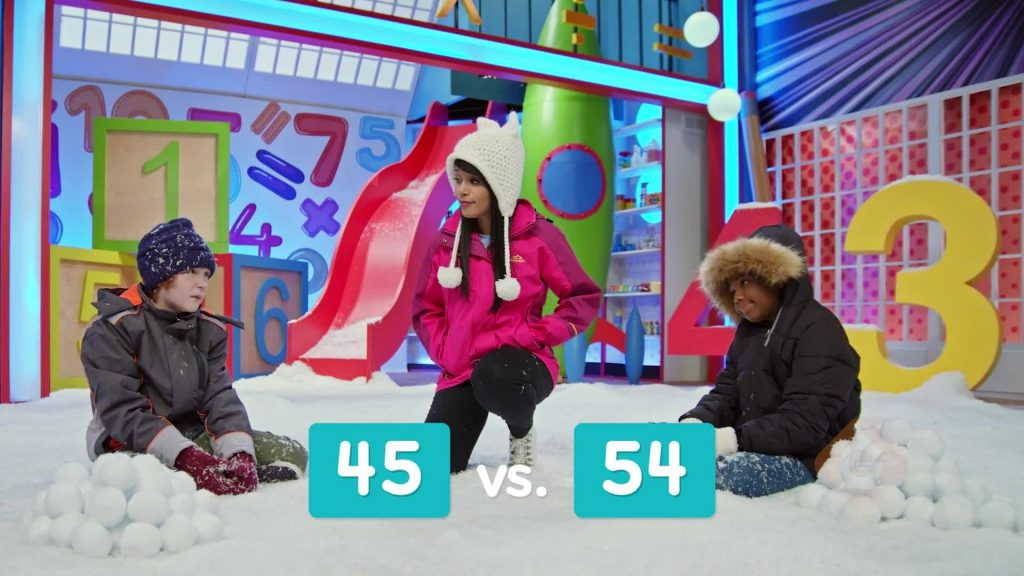WHAT IS COMPARING?

You can compare numbers to see which number is greater. You look at the digits and the place each digit is in. You decide if one number is less than (<), equal to (=), or greater than (>) another number.

To better understand comparing 2-digit numbers…

WHAT IS COMPARING?. You can compare numbers to see which number is greater. You look at the digits and the place each digit is in. You decide if one number is less than (<), equal to (=), or greater than (>) another number. To better understand comparing 2-digit numbers…

## LET’S BREAK IT DOWN!

### Compare the number of tickets.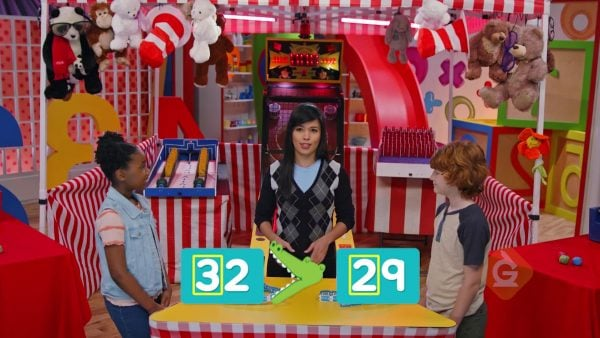Gavin has 32 tickets. Amiyah has 29 tickets. 32 has 3 tens. 29 has 2 tens. 32 has more tens than 29. 32 is greater than 29. 32 > 29.

Compare the number of tickets. Gavin has 32 tickets. Amiyah has 29 tickets. 32 has 3 tens. 29 has 2 tens. 32 has more tens than 29. 32 is greater than 29. 32 > 29.

### Compare the number of stickers.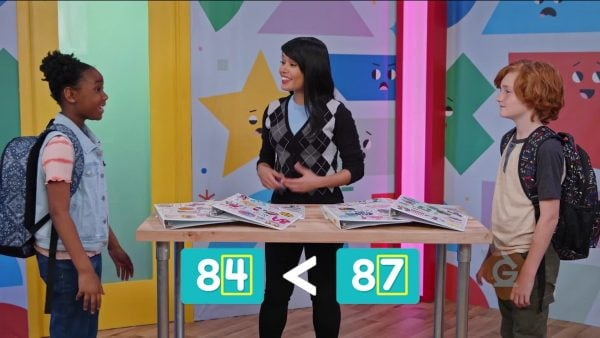Amiyah has 84 stickers. Gavin has 87 stickers. 84 and 87 have the same number of tens. 87 has more ones than 84. 87 is greater than 84. 87 > 84.

Compare the number of stickers. Amiyah has 84 stickers. Gavin has 87 stickers. 84 and 87 have the same number of tens. 87 has more ones than 84. 87 is greater than 84. 87 > 84.

### How to know when two numbers are equal.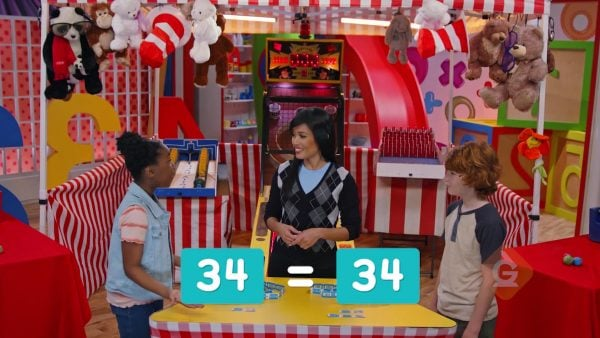Amiyah has 34 tickets and Gavin has 34 tickets. There are 3 tens in each number of tickets. There are 4 ones in each number. 34 is equal to 34. 34 = 34.

How to know when two numbers are equal. Amiyah has 34 tickets and Gavin has 34 tickets. There are 3 tens in each number of tickets. There are 4 ones in each number. 34 is equal to 34. 34 = 34.

### 45 and 54 are not equal.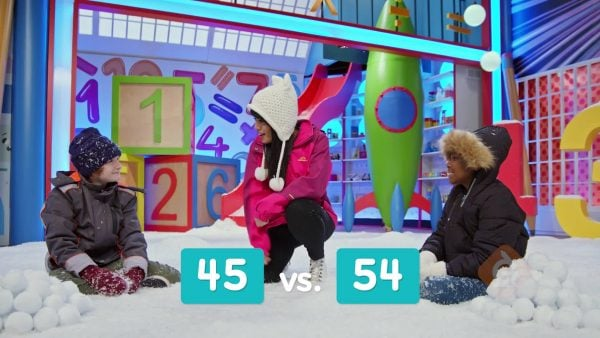45 and 54 have the same digits. But the digits are not in the same place. 54 has 5 tens. 45 has 4 tens. 45 has fewer tens than 54. 45 is less than 54. 45 < 54.

45 and 54 are not equal. 45 and 54 have the same digits. But the digits are not in the same place. 54 has 5 tens. 45 has 4 tens. 45 has fewer tens than 54. 45 is less than 54. 45 < 54.

### There are two ways to compare numbers.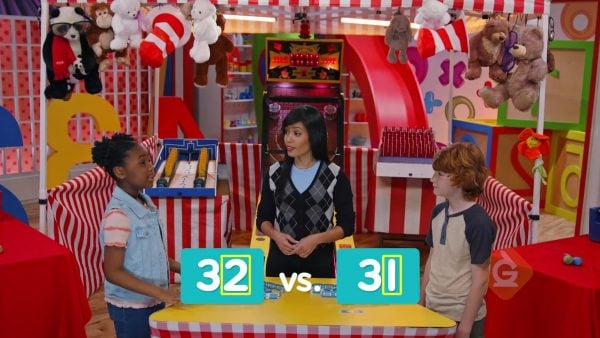There are two ways to show how 32 and 31 compare. 31 is less than 32: 31 < 32. 32 is greater than 31: 32 > 31.

There are two ways to compare numbers. There are two ways to show how 32 and 31 compare. 31 is less than 32: 31 < 32. 32 is greater than 31: 32 > 31.

## COMPARING 2-DIGIT NUMBERS VOCABULARY

Compare numbers
Look at a number to see if it is greater than, equal to, or less than another number.
2-digit number
A number that has two digits, one in the tens place and one in the ones place.
Place value
The value of a digit based on its position in a number.
Greater than sign (>)
Sign used to show one number is more/greater than another number.
Less than sign (<)
Sign used to show one number is less than another number.
Equal sign (=)
Sign used to show that one number has the same value as another number.
Blocks used to help students understand place value.
The place in a number where the digit that tells the number of tens goes.
The place in a number where the digit that tells the number of ones goes.
The place in a number that has the highest value. In a 2-digit number, the tens place is the greatest place.

## COMPARING 2-DIGIT NUMBERS DISCUSSION QUESTIONS

### Use <, =, or > to compare 37 and 42. Explain how you compared.

37 has 3 tens. 42 has 4 tens. 42 has more tens. 42 > 37 or 37 < 42

25 has two tens. 29 has two tens. The digits in tens place are the same. So, we compare the ones place. 25 has 5 ones. 29 has 9 ones. 9 is greater than 5, so 29 > 25. Onawa read more books.

### Use <, =, or > to compare 53 red marbles and 53 blue marbles. Explain.

The number of red marbles, 53, has 5 tens and 3 ones. The number of blue marbles, 53, also has 5 tens and 3 ones. 53 = 53

### Tyree says that 56 = 65 because each number has the digit 5 and the digit 6 in it. Is Tyree correct? Explain.

Tyree is not correct. You have to look at which place each digit is in. 65 has 6 tens. 56 has 5 tens. 6 is greater than 5. 65 > 56

### Jessica wrote 23 > 19 when she compared 23 and 19. What is another way to show how those numbers compare?

It is true that 23 is greater than 19. It is also true that 19 is less than 23. 19 < 23
X

## Success

We’ve sent you an email with instructions how to reset your password.
Ok